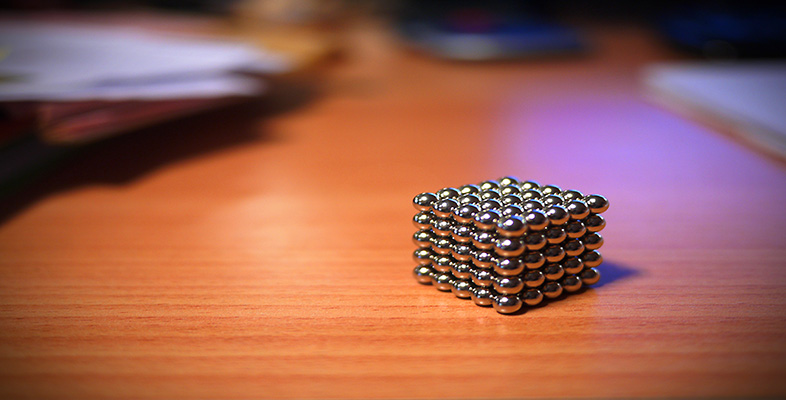Squares, roots and powers

This free course is available to start right now. Review the full course description and key learning outcomes and create an account and enrol if you want a free statement of participation.

Free course

2.3.1 Try some yourself

Activity 22

Write the following as one number to a single power:

• (a) 23 × 24

• (b) 32 × 34

• (c) 42 × 43 × 44

• (a) 23 × 24 = 2(3 + 4) = 27

• (b) 32 × 34 = 3(2 + 4) = 36

• (c) 42 × 43 × 44 = 4(2 + 3 + 4) = 49

Activity 23

Without using your calculator, find the following, as estimates for calculation work in the next question.

• (a) (1)5

• (b) 34

• (a) (1)5 = 1 (any odd power of 1 is 1)

• (b) 34 = 3 × 3 × 3 × 3 = 81

Activity 24

Use your calculator to find the following.

• (a) (1.3)5

• (b) 3.24

• (a) (1.3)53.7129

• (b) 3.24 = 104.8576

MU120_4M4